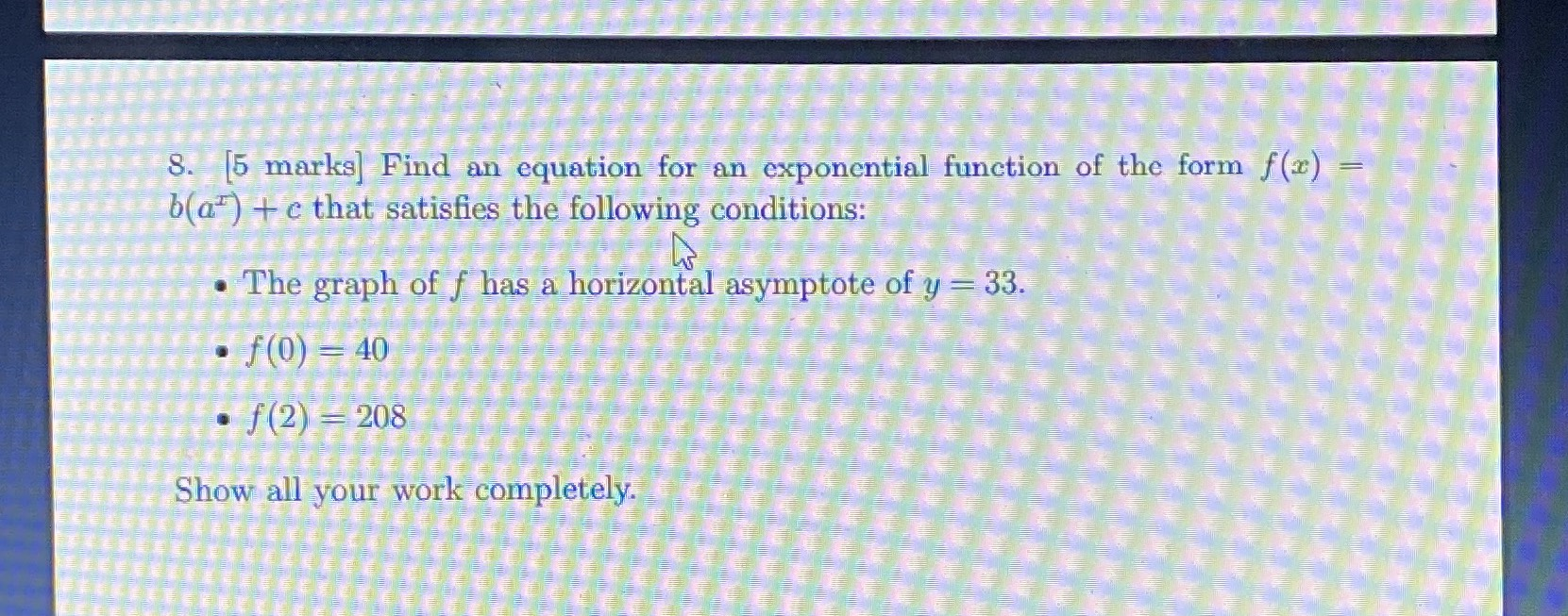### Still have math questions?

Algebra
Question8. [5 marks] Find an equation for an exponential function of the form $$f ( x ) =$$ $$b ( a ^ { x } ) + c$$ that satisfies the following conditions:

-The graph of $$f$$ has a horizontal asymptote of $$y = 33$$ .

$$f ( 0 ) = 40$$

$$f ( 2 ) = 208$$

$$f ( x ) = 7 ( 5 ) ^ { x } + 33$$ or $$f ( x ) = 7 ( - 5 ) ^ { x } + 33$$